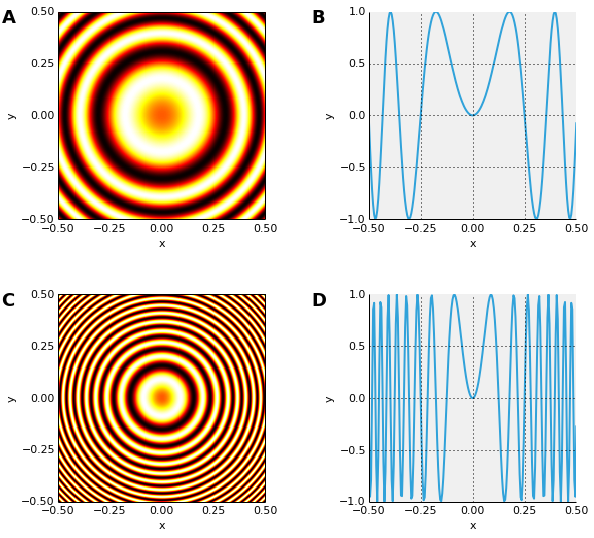CoCalc Public Filessupport / 2015-03-19-111401-holoviews.ipynb
Description: Jupyter notebook support/2015-06-04-141749-bokeh.ipynb
In :
from holoviews import *
from holoviews.operation import contours, threshold, gradient
import numpy as np


In :
def sine(x, phase=0, freq=100):
return np.sin((freq * x + phase))

In :
phases = np.linspace(0,2*np.pi,11) # Explored phases
freqs = np.linspace(50,150,5)      # Explored frequencies

In :
dist = np.linspace(-0.5,0.5,202)   # Linear spatial sampling
x,y = np.meshgrid(dist, dist)
grid = (x**2+y**2)                 # 2D spatial sampling

In :
freq1 = Image(sine(grid, freq=50))  + Curve(zip(dist, sine(dist**2, freq=50)))
freq2 = Image(sine(grid, freq=200)) + Curve(zip(dist, sine(dist**2, freq=200)))
(freq1 + freq2).cols(2)In [ ]: